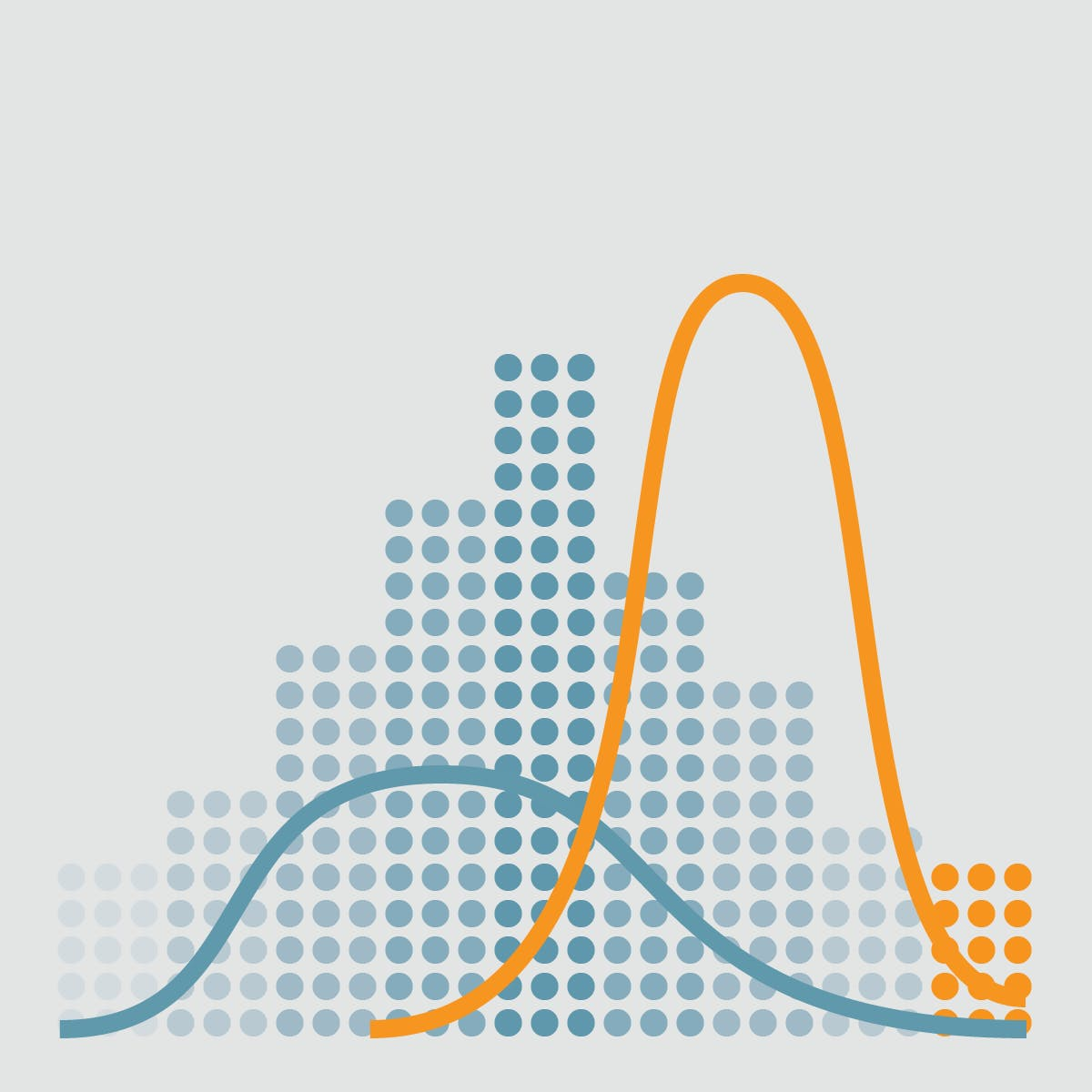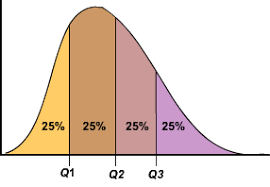# 2.5 - Estimates of Dispersion

Last updated: March 15th, 2019# Estimates of Dispersion¶

Location is just one dimension in summarizing a feature. A second dimension, dispersion, also referred to as variability, measures whether the data values are tightly clustered or spread out.## Hands on!¶

In [ ]:
import numpy as np
import pandas as pd
import matplotlib.pyplot as plt
import seaborn as sns

%matplotlib inline


We'll use the following Google Play Store Apps dataset in this lesson:

In [ ]:
df = pd.read_csv('data/googleplaystore.csv')

df.head()### Variance and Standard Deviation¶

The best-known estimates for variability are the variance and the standard deviation, which are based on squared deviations between the estimate of location and the observed data.

The variance is an average of the squared deviations:

$$Variance = s^2 = \frac{\sum\limits_{i}^n (x_i - \overline{x})^2 }{n-1}$$

We can easily calculate it in pandas with the var() method:

In [ ]:
df['Rating'].var()


The standard deviation is the square root of the variance, and is much easier to interpret than the variance since it is on the same scale as the original data.

$$Standard \ deviation = s = \sigma = \sqrt{Variance}$$

Let's calculate both for the Rating of Google Play Store Apps: We can calculate the Standard Deviation as the square root of the variance, or directly (and preferably) with the std() method:

In [ ]:
import math

math.sqrt(df['Rating'].var())

In [ ]:
df['Rating'].std()


Both variance and standar deviation are especially sensitive to outliers since they are based on the squared deviations.

In [ ]:
plt.figure(figsize=(12,6))

sns.distplot(df['Rating'].dropna())

# Mean line
plt.axvline(df['Rating'].mean(), color='#e74c3c', linestyle='dashed', linewidth=2)

# Standard deviation lines
plt.axvline(df['Rating'].mean() + df['Rating'].std(), color="#2c3e50", linestyle='dotted', linewidth=2)
plt.axvline(df['Rating'].mean() - df['Rating'].std(), color="#2c3e50", linestyle='dotted', linewidth=2)### Estimates based on percentiles¶

Any set of data, arranged in ascending or descending order, can be divided into various parts, also known as partitions or subsets, regulated by quantiles. Quantile is a generic term for those values that divide the set into partitions of size n, so that each part represents 1/n of the set. Quantiles are not the partition itself, they are the numbers that define the partition. You can think of them as a sort of numeric boundary.

#### Percentiles¶

A percentile is a measure at which that percentage of the total values are the same as or below that measure. For example, 90% of the data values lie below the 90th percentile, whereas 10% of the data values lie below the 10th percentile.

$$percentile(n) = \frac{number\ of\ values\ below\ n}{size\ of\ set\ x} * 100$$

#### Quartiles¶

Quartiles are values that divide a (part of a) data table into four groups containing an approximately equal number of observations. The total of 100% is split into four equal parts: 25%, 50%, 75% and 100%.

The first quartile (or lower quartile), Q1, is defined as the value that has an f-value equal to 0.25. This is the same thing as the twenty-fifth percentile.

The second quartile always corresponds to the median of the set x.

The third quartile (or upper quartile), Q3, has an f-value equal to 0.75.

The interquartile range, IQR, is defined as Q3-Q1.In [ ]:
df['Rating'].mean()

In [ ]:
df['Rating'].median()

In [ ]:
df['Rating'].quantile(0.5)

In [ ]:
quartiles = df['Rating'].quantile([0.25, 0.5, 0.75, 1])

quartiles

In [ ]:
plt.figure(figsize=(12,6))

sns.distplot(df['Rating'].dropna())

# Median line
plt.axvline(df['Rating'].median(), color='#e74c3c', linestyle='dashed', linewidth=2)

for i, q in enumerate(quartiles):
# Quartile i line
plt.axvline(q, color='#27ae60', linestyle='dotted', linewidth=2)

In [ ]:
percentiles = df['Rating'].quantile(np.arange(0.1, 1.1, 0.1))

percentiles

In [ ]:
plt.figure(figsize=(12,6))

sns.distplot(df['Rating'].dropna())

# Median line
plt.axvline(df['Rating'].median(), color='#e74c3c', linestyle='dashed', linewidth=2)

for i, q in enumerate(percentiles):
# Percentile i line
plt.axvline(q, color='#27ae60', linestyle='dotted', linewidth=2)